You are here: Start » AVL.NET » Geometry 2D Intersections

# Geometry 2D Intersections

Icon Name Description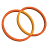CircleCircleIntersection Computes common points of two circles.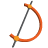LineArcIntersection Computes the common points of an arc and a line.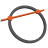LineCircleIntersection Computes common points of a circle and a line.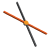LineLineIntersection Computes a common point of two lines.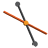LineSegmentIntersection Computes a common point of a line and a segment.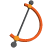SegmentArcIntersection Computes the common points of an arc and a segment.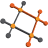SegmentArrayIntersections Computes a common points or segment of any segments from the set.SegmentCircleIntersection Computes the common points of a circle and a segment.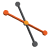SegmentSegmentIntersection Computes a common point of two segments.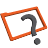TestRectangleIntersectsWith Tests if two rectangles intersect.